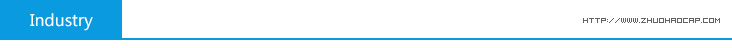Welcome to Dongguan Zhuo Wang Electronics Technology Co., Ltd. official website!Product Categories#### How to detect electrolytic capacitors?

1) After measuring the electrolytic capacitor leakage resistance range selection in accordance with the method described above, select the appropriate range multimeter, the red pen then negative, then positive black pen, this time, hands swinging in the direction of zero to R, placed in a certain range , and the reverse direction to the dollar poor swing, until a position to stop, resistance at this time the pointer is positive leakage resistance, the greater the positive leakage resistance, indicating better performance capacitor leakage current is more small. The multimeter red, black pen swap (red pen then positive, then negative black pen), then measure the resistance point the pointer to reverse leakage resistance of the capacitor, this value should be smaller than the forward leakage resistance . As measured two drain resistance value is very small (a few hundred kilohms or less), then the poor performance of the electrolytic capacitor can not be used. Detection method for electrolytic capacitor
Because the capacity of the electrolytic capacitor is much greater than the average fixed capacitance, so measurements should be used in the appropriate range for different capacity. According to experience, in general, 1 ~ 47μF capacitance between the available R × 1k block measurement, the capacitance is greater than the 47μF R × 100 block measuring.
For positive and negative signs of unknown electrolytic capacitor, can be measured leakage resistance is determined using the above method. That is, first measured about any leakage resistance, remember its size, and then swap out a pen and then measure the resistance. Two large resistance measurements that time would be a positive connection, namely the black pen then is positive, the red pen then is negative.

2) electrolytic capacitor positive and negative electrodes of the electrolytic capacitor determining the positive and negative electrode mainly discrimination method of the measurement method according to the above leakage resistance. With a multimeter ohm block, according to the capacity of the electrolytic capacitor selected the appropriate range, with two pen then two pin capacitor leakage test its resistance, and note that the size of the resistance, and then the two swapped leads once again measured leakage resistance value, the leakage resistance value comparison of the two measurements, the leakage resistance of a small, black pen is in contact with the anode electrolytic capacitor.
Capacitor with a multimeter for testing should pay attention to the following three points:
① whether the capacitor leakage resistance measurements, or short circuit measurements, the measurement process should pay attention to the hand can not touch the two leads.
② Since the capacitor in the measurement process to have the charge and discharge process, so when the first measurement, you must first discharge (with a multimeter test leads to what can be short-circuited capacitor two leads), and before the second measurement.
③ way to detect when a capacitor, we must clarify whether other components of the circuit where the influence of the measurement results, in general, should try not to use the road measurements.